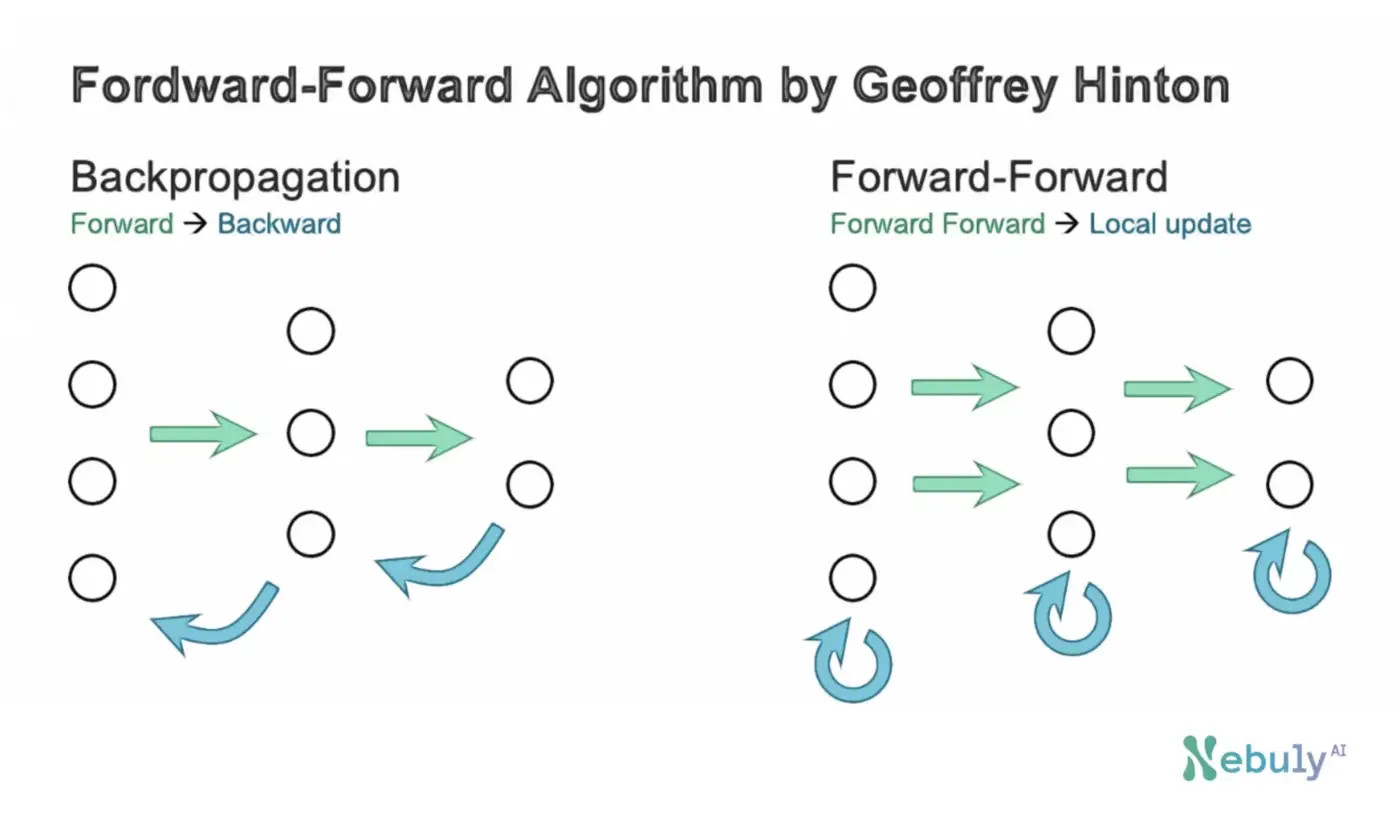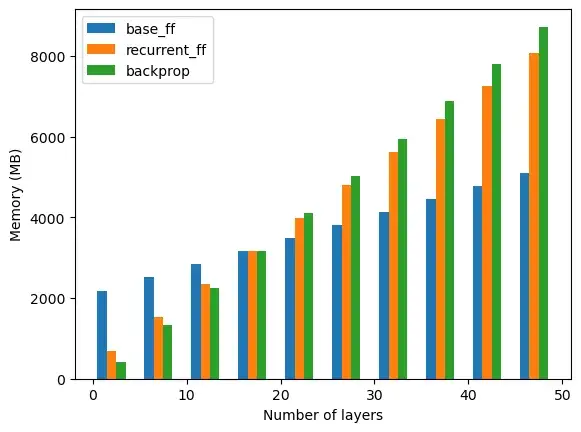# PyTorch implementation of Geoffrey Hinton’s Forward-Forward algorithmImage by Nebuly

Are you an AI researcher itching to test Geoffrey Hinton’s Forward-Forward Algorithm? I was too, but could not find any full implementation so I decided to code it myself, from scratch. Good news — you can access the full PyTorch implementation on GitHub here. Don’t forget to leave a star if you enjoy the project ⭐

As soon as I read the paper, I started to wonder how AI stands to benefit from Hinton’s FF algorithm (FF = Forward-Forward). I got particularly interested in the following concepts:

## Key insights on Forward-Forward memory usage

• Local training. Each layer can be trained just comparing the outputs for positive and negative streams.
• No need to store the activations. Activations are needed during the backpropagation to compute gradients, but often result in nasty Out of Memory errors.
• Faster weights layer update. Once the output of a layer has been computed, the weights can be updated right away, i.e. no need to wait the full forward (and part of the backward) pass to be completed.
• Alternative goodness metrics. Hinton’s paper uses the sum-square of the output as goodness metric, but I expect alternative metrics to pop up in scientific literature over the coming months.

Hinton’s paper proposed 2 different Forward-Forward algorithms, which I called Base and Recurrent. Let’s examine the 3 key aspects of the performance of these algorithms compared with their alternative, backpropagation.Performance comparison of backprop vs Base and Recurrent Forward-Forward. Image by Nebuly

### 1. Base Forward-Forward memory usage increases with the number of layers

The first interesting insight is that the memory usage of the Forward-Forward algorithm still increases with respect to the number of layers, but significantly less with respect to the backpropagation algorithm. This is due to the fact that the increase in memory usage for the Forward-Forward algorithm is just related to the number of parameters of the network: each layer contains 2000x2000 parameters which when trained using the Adam optimizer occupies approximately 64 MB. The total memory usage difference between n_layers=2 and n_layers=47 is approximately 2.8 GB which corresponds to 64MB * 45 layers.

### 2. Base Forward-Forward has a worse memory usage than backprop for thin models

From the plot, we see that for few layers the Forward-Forward algorithm occupies much more memory than the backprop counterpart (around 2GB vs 400MB). This can be partially explained by considering the structure of the Forward-Forward algorithm. For FF, we need to replicate each input by the number of possible classes (10 in MNIST), and this means that the effective batch size becomes 10x with respect to the original one. Let’s now do the math: when evaluating the network, we give the model the whole validation set in a unique batch (10’000 images). Considering a hidden dimension of 2000, the memory occupied by each hidden state is 80 MB (we run the model with 32-bit precision). This means that the effective batch size becomes 100’000 images and the memory occupied during inference is approximately 800MB. This quick calculation already shows the higher memory usage by FF compared to backprop for thin models, but it does not yield the 2 GB-plus results obtained during testing. Further investigation is needed to explain FF’s exact memory usage.

### 3. Recurrent FF does not have great memory usage advantages

Unlike Base FF, Recurrent FF does not have a clear memory advantage versus backprop for deep networks (15+ layers). That’s by design since the recurrent network must save each intermediate step at time t to compute the following and previous layer outputs at time t+1. While scientifically relevant, the Recurrent FF is clearly less performant memory-wise than the Base FF.

#### What's next?

The Forward-Forward algorithm could in practice be further optimized as it does not require loading the full network while training. In fact, the Forward-Forward algorithm can be used to train each layer of the network separately, meaning that the memory usage of the algorithm would be related just to the number of parameters of the layer being trained.